# 1-Fat Ring Graph with 200 vertices

The Second DIMACS Implementation Challenge: 1992-1993

Originator: Panos Pardalos

NP Hard Problems: Maximum Clique, Graph Coloring, and Satisfiability, The Second DIMACS Implementation Challenge: 1992-1993

(200 vertices, 1534 edges)

## Examples

### Basic Examples

Retrieve the graph:

 In:=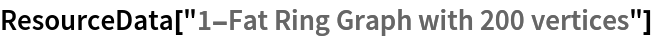Out=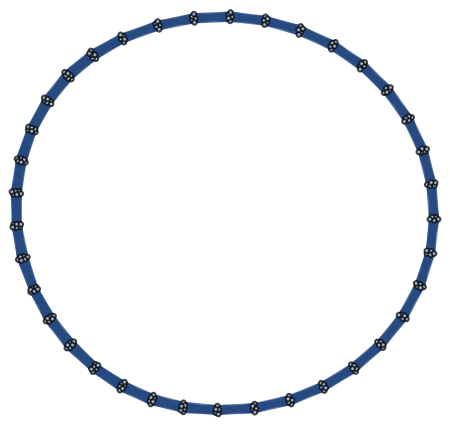Summary properties:

 In:=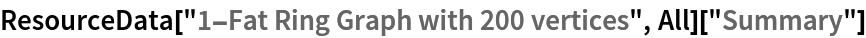Out=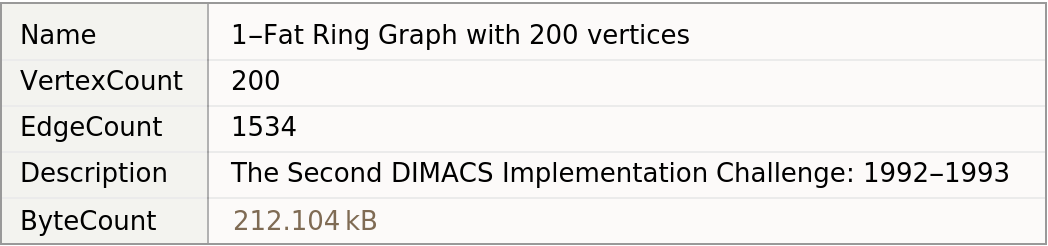### Basic Applications

Find the maximum clique:

 In:=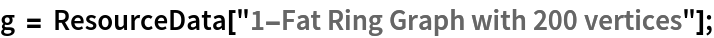In:=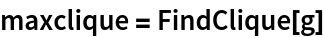Out=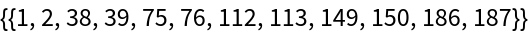Show the maximum clique:

 In:=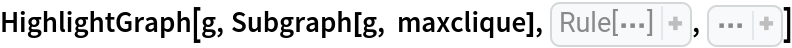Out=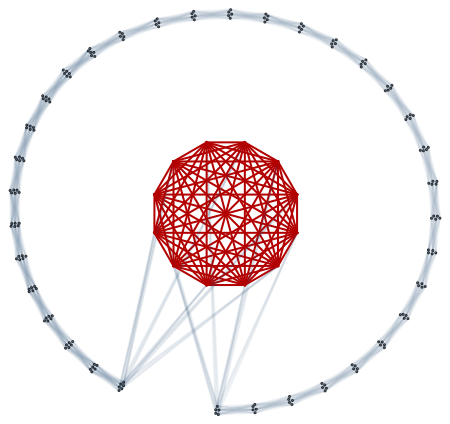Wolfram Research, "1-Fat Ring Graph with 200 vertices" from the Wolfram Data Repository (2019)